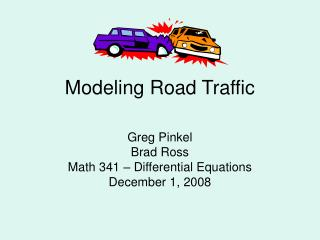DownloadDownload PresentationTélécharger la présentation- - - - - - - - - - - - - - - - - - - - - - - - - - - E N D - - - - - - - - - - - - - - - - - - - - - - - - - - -
##### Presentation Transcript

1. Modeling Road Traffic Greg Pinkel Brad Ross Math 341 – Differential Equations December 1, 2008

2. Contents • Introduction • Model • Revised Model • Solving our model • Conclusions

3. Introduction • Modeling the behavior of cars in traffic • Car Following Model • Quick-Thinking-Driver (QTD) Car Following Model • Major Results: • If lead car stops suddenly, system looks like a damped harmonic oscillator • Temporal Following Spacing should be ≥ 2 sec

4. Overview • Purpose – traffic modeling • Models • 2-car model, x0 position of leading car, x1position of following car (meters) • Acceleration of following car (m/s2) • Sensitivity coefficients, λ (1/sec.) and β (1/sec.2) • Reaction time T (seconds) • Preferred separation, d (meters) or temporal separation, τ (seconds) OriginalModel Quick Thinking Driver Model

5. Original Model • λ : sensitivity coefficient, units s-1 • Impact of and • Addition of T is a Delay Differential Equation, very difficult to solve analytically

6. Quick Thinking Driver Model • Ignores reaction time for driver • β : sensitivity coefficient, units s-2 • Assuming d is a constant “preferred separation” • Impact of x0 – x1<d and x0 – x1>d

7. Preferred Separation • Tends to depend on speed (Think of real world situation) • Relate preferred spatial separation to temporal separation by • with a preferred temporal separation of τ seconds.

8. Physically Reasonable Stopping With initial conditions , D = Separation of vehicles, x0 –x1, at time of x0 sudden stop m m/s

9. Solving: Laplace Transforms and Mathematica!

10. The Solution: X1(t)

11. Major Results: Simple Harmonic Motion • Have three distinct cases for different values of τ: Overdamped, (τ=1s) Underdamped, (τ=3s) Critically Damped, (τ=2s) U=10 m/s, D=10m, β=1s-2

12. Significance of Results • Always have collision with the underdamped situation •  • To avoid collision: τ2 ≥ 4, τ ≥ 2 seconds

13. Minimum Stopping Distance • Collision is possible but will not necessarily happen: a βτ2 > 4, βτ2 = 4 • Collision can be avoided by ensuring initial separation greater than Dstop • Derived by setting the solution of x(t) equal to zero with given conditions, D becomes Dstop • Having initial separation greater than this ensures that the collision will be avoided

14. Conclusion • Based on conditions: βτ2 > 4, βτ2 = 4, and βτ2 < 4 • collision is only avoidable for βτ2 ≥ 4 • collision only avoided ifx0 – x1 > Dstop • Because βτ2 < 4 always results in collision, maintain temporal time cushion at least 2 sec behind lead car